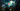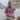# When 'Not' to Use Arrow Functions

Updated July 18, 2019Start discussion

It is a pleasure to see the evolution of the programming language you code every day. Learning from mistakes, searching for better implementation, creating new features is what makes the progress from version to version.

This is happening to JavaScript these years when ECMAScript 6 brings the language to a new level of usability: arrow functions, classes and a lot more. And this is great!

One of the most valuable new features is the arrow function. There are plenty of good articles that describe its context transparency and short syntax. If you’re new to ES6, take a start from reading about it.

But every medal has two sides. Often new features introduce some confusion, one of which is the arrow functions misguided utilization.

This article guides through scenarios where you should bypass the arrow function in favor of good old function expressions or newer shorthand method syntax. And take precautions with shortening, because it can affect the code readability.

## 1. Defining methods on an object

In JavaScript, the method is a function stored in a property of an object. When calling the method, `this` becomes the object that the method belongs to.

### 1a. Object literal

Since arrow function has a short syntax, it’s inviting to use it for a method definition. Let’s take a try:

``````const calculate = {
array: [1, 2, 3],
sum: () => {
console.log(this === window); // => true
return this.array.reduce((result, item) => result + item);
}
};
console.log(this === window); // => true
// Throws "TypeError: Cannot read property 'reduce' of undefined"
calculate.sum();``````

`calculate.sum` method is defined with an arrow function. But on invocation `calculate.sum()` throws a `TypeError`, because `this.array` is evaluated to `undefined`.
When invoking the method `sum()` on the `calculate` object, the context remains `window`. It happens because the arrow function binds the context lexically with the `window` object.
Executing `this.array` is equivalent to `window.array`, which is `undefined`.

The solution is to use a function expression or shorthand syntax for method definition (available in ECMAScript 6). In such a case `this` is determined by the invocation, but not by the enclosing context. Let’s see the fixed version:

``````const calculate = {
array: [1, 2, 3],
sum() {
console.log(this === calculate); // => true
return this.array.reduce((result, item) => result + item);
}
};
calculate.sum(); // => 6``````

Because `sum` is a regular function, `this` on invocation of `calculate.sum()` is the `calculate` object. `this.array` is the array reference, therefore the sum of elements is calculated correctly: `6`.

### 1b. Object prototype

The same rule applies when defining methods on a `prototype` object.
Instead of using an arrow function for defining `sayCatName` method, which brings an incorrect context `window`:

``````function MyCat(name) {
this.catName = name;
}
MyCat.prototype.sayCatName = () => {
console.log(this === window); // => true
return this.catName;
};
const cat = new MyCat('Mew');
cat.sayCatName(); // => undefined``````

use the old school function expression:

``````function MyCat(name) {
this.catName = name;
}
MyCat.prototype.sayCatName = function() {
console.log(this === cat); // => true
return this.catName;
};
const cat = new MyCat('Mew');
cat.sayCatName(); // => 'Mew'``````

`sayCatName` regular function is changing the context to `cat` object when called as a method: `cat.sayCatName()`.

## 2. Callback functions with dynamic context

`this` in JavaScript is a powerful feature. It allows changing the context depending on the way a function is called. Frequently the context is the target object on which invocation happens, making the code more natural. It says like “something is happening with this object”.

However, the arrow function binds the context statically on the declaration and is not possible to make it dynamic. It’s the other side of the medal in a situation when lexical `this` is not necessary.

Attaching event listeners to DOM elements is a common task in client-side programming. An event triggers the handler function with `this` as the target element. Handy usage of the dynamic context.

The following example is trying to use an arrow function for such a handler:

``````const button = document.getElementById('myButton');
console.log(this === window); // => true
this.innerHTML = 'Clicked button';
});``````

`this` is `window` in an arrow function that is defined in the global context. When a click event happens, browser tries to invoke the handler function with `button` context, but arrow function does not change its pre-defined context.
`this.innerHTML` is equivalent to `window.innerHTML` and has no sense.

You have to apply a function expression, which allows to change `this` depending on the target element:

``````const button = document.getElementById('myButton');
console.log(this === button); // => true
this.innerHTML = 'Clicked button';
});``````

When user clicks the button, `this` in the handler function is `button`. Thus `this.innerHTML = 'Clicked button'` modifies correctly the button text to reflect clicked status.

## 3. Invoking constructors

`this` in a construction invocation is the newly created object. When executing `new MyFunction()`, the context of the constructor `MyFunction` is a new object: `this instanceof MyFunction === true`.

Notice that an arrow function cannot be used as a constructor. JavaScript implicitly prevents from doing that by throwing an exception.
Anyway `this` is setup from the enclosing context and is not the newly created object. In other words, an arrow function constructor invocation doesn’t make sense and is ambiguous.
Let’s see what happens if however trying to:

``````const Message = (text) => {
this.text = text;
};
// Throws "TypeError: Message is not a constructor"
const helloMessage = new Message('Hello World!');``````

Executing `new Message('Hello World!')`, where `Message` is an arrow function, JavaScript throws a `TypeError` that `Message` cannot be used as a constructor.

I consider an efficient practice that ECMAScript 6 fails with verbose error messages in such situations. Contrary to fail silently specific to previous JavaScript versions.

The above example is fixed using a function expression, which is the correct way (including the function declaration) to create constructors:

``````const Message = function(text) {
this.text = text;
};
const helloMessage = new Message('Hello World!');
console.log(helloMessage.text); // => 'Hello World!'``````

## 4. Too short syntax

The arrow function has a nice property of omitting the arguments parenthesis `()`, block curly brackets `{}` and `return` if the function body has one statement. This helps in writing very short functions.

My university professor of programming gives students an interesting task: write the shortest function that counts the string length in C language. This is a good approach to study and explore a new language.

Nevertheless, in real-world applications, the code is read by many developers. The shortest syntax is not always appropriate to help your colleague understand the function on the fly.

At some level the compressed function becomes difficult to read, so try not to get into passion. Let’s see an example:

``````const multiply = (a, b) => b === undefined ? b => a * b : a * b;
const double = multiply(2);
double(3);      // => 6
multiply(2, 3); // => 6``````

`multiply` returns the multiplication result of two numbers or a closure tied with first parameter for later multiplication.
The function works nice and looks short. But it may be tough to understand what it does from the first look.

To make it more readable, it is possible to restore the optional curly braces and `return` statement from the arrow function or use a regular function:

``````function multiply(a, b) {
if (b === undefined) {
return function(b) {
return a * b;
}
}
return a * b;
}
const double = multiply(2);
double(3);      // => 6
multiply(2, 3); // => 6``````

It is good to find a balance between short and verbose to make your JavaScript straightforward.

## 5. Conclusion

Without a doubt, the arrow function is a great addition. When used correctly it brings simplicity in places where earlier you had to use `.bind()` or trying to catch the context. It also makes the code lighter.

Advantages in some situations bring disadvantages in others. You can’t use an arrow function when a dynamic context is required: defining methods, create objects with constructors, get the target from `this` when handling events.

## Quality posts into your inbox

I regularly publish posts containing:

• Important JavaScript concepts explained in simple words
• Overview of new JavaScript features
• How to use TypeScript and typing
• Software design and good coding practices